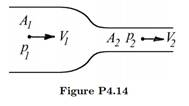# Consider a constant density gas flowing steadily through a smooth, circular, horizontal contraction. Calculate the velocity and pressure as the radius decreases to half its original value. State all your assumptions.

Question
1 views

Consider a constant density gas flowing steadily through a smooth, circular, horizontal contraction. Calculate the velocity and pressure as the radius decreases to half its original value. State all your assumptions.### This question hasn't been answered yet.